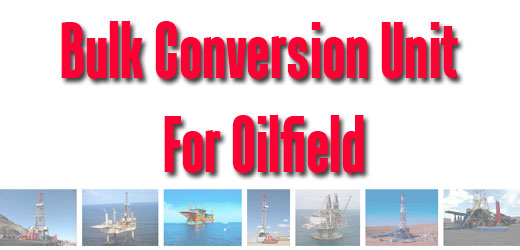## Bulk Conversion Unit for OilfieldI have heard people asking like this “How many sacks of barite are equal to 1 cubic of barite?”, “How much is weight of one sack of cement?”, “I receive 20 tons of bentonite. What is the factor to covert my bentonite in ton to sacks”, etc.Therefore, I collect several useful conversions used for bulk on the drilling rigs.The bulk conversion factors are listed below:

Fresh water

1 Gal (US) water = 0.83267 Gal (IMP)

1 Gal (US) water = 8.3453 lbs

1 Gal (US) water = 3.785 liters

1 liter of water = 2.2048 lbs

1000 liters of water = 2205 lbs

1 cubic meter of water = 2205 lbs

1 metric ton of water = 2205 lbs

1 metric ton of water = 264 Gal (US)

1 metric ton of water = 220 Gal (IMP)

1 metric ton of water = 6.29 BBL (US)

Diesel (Average Weight)

1 Gal (US) diesel = 7 lbs

1 Gal (IMP) diesel = 8.4 lbs

1 liter of diesel = 1.85 lbs

1 cubic meter of diesel = 1850 lbs

1 metric ton of diesel = 2205 lb

1 metric ton of diesel =315 Gal (US)

1 metric ton of diesel = 263 Gal (IMP)

1 metric ton of diesel = 1190 liters

Barite

1 sack of barite = 100 lb

1 cubic foot of barite = 135 lb

1 metric ton of barite = 2205 lb

1 metric ton of barite = 22 sacks

1 metric ton of barite =16.3 cubic foot

Bentonite

1 sack of bentonite = 100 lb

1 cubic foot of bentonite = 60 lb

1 metric ton of bentonite = 2205 lb

1 metric ton of bentonite = 22 sacks

1 metric ton of bentonite =36.8 cubic foot

Cement

1 sack of cement = 94 lb

1 sack of cement = 1 cubic foot

1 metric ton of cement = 2205 lb

1 metric ton of cement = 23.5 sacks

1 metric ton of cement =23.5 cubic foot

Gycol

1 Gal (US) glycol = 9.5 lb

1 Gal (IMP) glycol = 11.5 lb

1 liter glycol = 3.2 lb

Aviation Fuel (Average Weight)

1 Gal (US) aviation fuel = 6.8 lbs

1 Gal (IMP) aviation fuel = 8.2 lbs

1 liter aviation fuel = 1.8 lbs